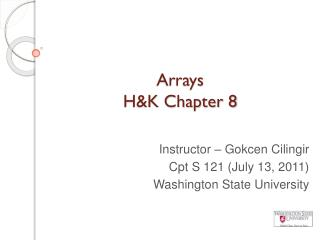DownloadDownload PresentationArrays H&K Chapter 8

# Arrays H&K Chapter 8

Télécharger la présentation## Arrays H&K Chapter 8

- - - - - - - - - - - - - - - - - - - - - - - - - - - E N D - - - - - - - - - - - - - - - - - - - - - - - - - - -
##### Presentation Transcript

1. Arrays H&K Chapter 8 Instructor – GokcenCilingir Cpt S 121 (July 13, 2011) Washington State University

3. What is an array? • An array is a data structure • A data structure is a way of storing and organizing data in memory so that it may be accessed and manipulated efficiently • An array is a sequence of items (of the same data type and of the same size) that are contiguously allocated in memory • Arrays are declared in much the same way as variables: int a; declares an array a with 6 cells that hold integers: Notice that array indexing begins at 0. a a a a a a

4. Declaring and manipulating arrays • We can declare arrays alongside simple variables: int students, count, teachers; double gpa, average; char ch, name; /*char arrays have a special name: string*/ • Assuming this array: all of the following statements are valid: a = 4; /* changes the value of a from 10 to 4 */ a += 2; /* sets the value of a to 2 */ a = a – a; /* sets the value of a to 88 */ a a a a a a

5. Array Subscripts • We can do arithmetic on array subscripts! Assume this array: Then all of the following are valid: int x = 2; printf("%d", a[x + 2]); /* a == 1 */ printf("%d", a[2 * x – 1]); /* a == 89 */ printf("%d", a[x] – a[x-1]); /* -12 */ printf("%d", a[++x]); /* a == 89; x == 3 */ a[x – 1] = a[x – 2]; /* assigns 12 to a */ printf("%d", a[x + 4]); /* Does a exist? */ a a a a a a

6. Initializing Arrays • We can initialize arrays at the time we declare them. Just as int count = 0; is valid, so too is intstudent_id[] = {3423, 8794, 4595, 1423, 4311, 5153, 9182, 1481, 1253, 1222,2521, 2251, 2111}; Notice how you can omit the size of the array; the compiler deduces the size from the number of values listed.

7. Initializing Arrays (2) • We can initialize arrays after we declared the array, using a loop: #define SIZE_LIMIT 100 int a[SIZE_LIMIT],i; for(i = 0; i < SIZE_LIMIT; i++) { a[i] = 0; } It’s common to define a constant that holds the max number of items we anticipate to store in an array Initializing array a by setting every cell of this array to zero

8. Example Problem statement: Write a segment of code that creates an array of 10 double values, populates the array with the values 1.0 through 10.0, and finally exchanges the 1st and 10th values.

9. Example – cont’d #define ARRAY_SIZE 10 int main(void) { double array[ARRAY_SIZE]; //double array[] = {1.0, 2.0, 3.0, 4.0, 5.0, // 6.0, 7.0, 8.0, 9.0, 10.0}; double value = 1.0, temp; inti = 0; //initializing the array for(i = 0; i < ARRAY_SIZE; i++) { grades[i] = value; value += 1.0; } //swapping first and last element temp = array; /*last element would be at index ARRAY_SIZE -1*/ array = array[ARRAY_SIZE -1]; array[ARRAY_SIZE -1] = temp; }

10. Motivation example - revisited • We often need to process each element of an array successively • Recall motivation example: Computing the difference from the mean for a group of grades • We can accomplish this with a counter loop that goes from 0 to one less than the array size, inclusive.

11. Motivation example solution (1) #include <stdio.h> #define SIZE_LIMIT 100 #define SENTINEL -1 void print_array (double arr[], int size); void print_differences (double arr[], int size, double mean); double calculate_mean (double arr[], int size); //assumed: user always enters double formattable input intmain (void) { inti = 0, size = 0; double grades[SIZE_LIMIT], mean = 0.0; printf("Please enter grades, and when you finish enter -1 to indicate that's the end of the grades (max 100 grades)\n"); //storing all the grades inputted in grades array i=0; scanf("%lf", &grades[i]); while(grades[i] != SENTINEL && i < SIZE_LIMIT){ i++; scanf("%lf", &grades[i]); } size = i; // set size of the array portion used

12. Motivation example solution (2) //control function, just to see if inputting part was successful //print_array(grades,size); //calculate&display mean mean = calculate_mean(grades,size); printf("Mean is %.2lf\n", mean); //print differences from mean print_differences(grades, i, mean); return 0; } void print_array(double arr[], int size) { inti = 0; for(i = 0; i< size; i++) printf("%.2lf ", arr[i]); printf("\n"); }

13. Motivation example solution (2) void printDifferences (double arr[], int size, double mean) { inti = 0; for(i = 0; i< size; i++) printf("%.2lf %.2lf\n", arr[i], arr[i] - mean); } double calculate_mean (double arr[], int size) { double mean = 0.0; inti=0; for(i = 0; i< size; i++) mean += arr[i]; mean /= size; return mean; }

14. Parallel Arrays (1) • Often, we'd like to associate the values in one array with those in another array • A list of student numbers, together with their class standings, for example • We can declare parallelarrays to accomplish this: #define NUM_STUDENTS 100 typedefenum {freshman, sophomore, junior, senior} class_t; intid[NUM_STUDENTS]; class_tclass[NUM_STUDENTS];

15. Parallel Arrays (2) • The parallel arrays of student numbers and class standings might look something like this: id id id id id id class class class class class class

16. References • J.R. Hanly & E.B. Koffman, Problem Solving and Program Design in C (6th Ed.), Addison-Wesley, 2010 • P.J. Deitel & H.M. Deitel, C How to Program (5th Ed.), Pearson Education , Inc., 2007.